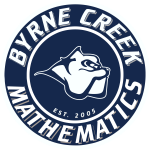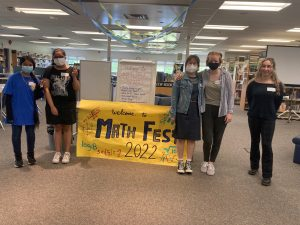# MATHEMATICSStaff Directory
 HNATIV, Maryana Maryana.Hnativ@burnabyschools.ca HUGHES, Sandra Sandra.Hughes@burnabyschools.ca Department Head LEE, Peter Peter.Lee@burnabyschools.ca LEE, Sharon Sharon.Lee@burnabyschools.ca STRUTHERS, Louise Louise.Struthers@burnabyschools.ca

Pathways

WORKPLACE MATHEMATICS
This pathway is designed to provide students with the mathematical understanding and critical thinking skills identified for entry into some trades and for direct entry into the workforce.

FOUNDATIONS MATHEMATICS
This pathway is designed to provide students with the mathematical understanding and critical thinking skills identified for post-secondary studies in programs that do not require the study of theoretical calculus (typically the Arts and Humanities.) *Foundations Math is only available at the grade 11 and 12 level.

PRE-CALCULUS & CALCULUS MATHEMATICS
This pathway is designed to provide students with the mathematical understanding and critical thinking skills identified for entry into post-secondary programs that require the study of calculus such as Sciences, Engineering, and Commerce.

Course Descriptions

MATHEMATICS 8
This is a year of consolidating basic mathematics skills; therefore, students must master all the learning outcomes. The course will provide students with the mathematical understandings and critical thinking skills so that they will be successful in future mathematics courses. Topics include: percent, proportional reasoning, powers and roots, rational numbers, Pythagorean Theorem, surface area and volume, discrete linear relations, two step equations, basic probabilities and statistics, and financial literacy. Problem solving will be incorporated into lessons throughout the year. In addition, algebra will be introduced at this level.

MATHEMATICS 9
This course introduces the topics that develop the framework for senior level courses in mathematics. The course will provide students with the mathematical understandings and critical thinking skills so that they will be successful in future mathematics courses. Topics include: operations with rational numbers, exponents, polynomials, linear relations, multi-step one variable equations, spatial proportional reasoning, statistics in society, and financial literacy. Problem solving will be incorporated into lessons throughout the year.

WORKPLACE MATHEMATICS 10
This course provides students with the mathematical understandings and critical thinking skills identified for entry into the majority of trades at post-secondary and for direct entry into the work force. The Workplace Math curriculum allows students to explore the logic of mathematics through real-life applications of math. Topics include: computational fluency, interpreting and constructing graphs, primary trigonometric rations, metric and imperial measurement and conversions, solving problems involving surface area and volume, angles, experimental probability, measures of central tendency, and financial literacy.

FOUNDATIONS & PRE-CALCULUS MATH 10
This course will prepare students for entry to either of the Foundation of Mathematics pathway or Pre-Calculus pathway starting in grade 11. The course will provide students with the mathematical understandings and critical thinking skills so that they will be successful in future mathematics courses. Topics include: powers with integral exponents, prime factorization, functions and relations, linear relations, arithmetic sequences, solving systems of linear equations, polynomials, right angled trigonometry, and financial literacy. Problem solving will be incorporated into lessons throughout the year.

WORKPLACE MATHEMATICS 11
Workplace Math 11 continues to develop the mathematical skills necessary for employment in the trades and general workplace. This curriculum allows students to explore the logic of mathematics through real-life applications of math. Topics include: computational fluency (through puzzles and games), statistics, 3D objects, linear relationships, slope as a rate of change, and financial literacy.

*Note: Students who successfully complete Workplace Math 11 have fulfilled their requirements for graduation.

FOUNDATIONS OF MATHEMATICS 11
This course is available to all students who have completed Mathematics 10. This course will prepare students for entry to post-secondary programs which do not require Pre-Calculus (typically the arts, humanities or social sciences). The course will provide students with the mathematical understandings and critical thinking skills so that they will be successful in future mathematics courses. Topics include: mathematical reasoning, relationships of angles, graphical representations of quadratic functions, graphical solutions to systems of equations, solving systems of linear inequalities, trigonometry, applications of probabilities and statistics, mathematics in research, and financial literacy.

PRE-CALCULUS 11
This is the second course of the Pre-Calculus pathway that further develops the mathematical skills necessary for the study of Calculus. It is intended for students whose future program of study will include Calculus. Topics include: real number system, powers with rational exponents, quadratic functions and equations, operations with rational and radical expressions, solving quadratic, rational and radical equations, polynomial factoring, linear and quadratic inequalities, trigonometry, and financial literacy.

FOUNDATIONS OF MATHEMATICS 12
This is the third course of the Foundations pathway that further develops the mathematical skills necessary for post-secondary studies in areas that do not require the study of Calculus. In Foundations of Mathematics 12 students will explore topics such as: logical reasoning, probability and its application, permutations, combinations, functions (polynomial, exponential, logarithmic, and sinusoidal), and geometry and spatial relationships.
*Students may take Foundations 12 and Pre-Calculus 12 concurrently.

PRE-CALCULUS 12
This is the third course of the Pre-Calculus pathway that further develops the mathematical skills necessary for the study of Calculus. This course is necessary to enter most post-secondary Science, Business and Engineering programs, with many requiring or recommending Calculus 12 as well. Topics include: trigonometry, transformations, exponential and logarithmic functions, rational functions, geometric sequences and series, and polynomials.

AP CALCULUS 12/CALCULUS 12
Calculus is an advanced mathematics course and is designed for students who are planning to pursue post-secondary studies in areas such as Sciences, Mathematics, Engineering and Commerce. Students should have a thorough understanding of the concepts introduced in Pre-Calculus 11 before enrolling in Calculus 12. Topics explored include: History of calculus, functions, graphs and limits, the derivative (concept and interpretations), the derivative (computing), applications of derivatives and graphing, applied problems and derivatives, integration, applications of integration. Students enrolled in AP Calculus may take the Advanced Placement exam, which can grant them one semester of math credit at many major universities. Only students writing the Advanced Placement exam will receive the AP Calculus designation in addition to Calculus 12 on their transcript.
* Calculus 12 may not be taken in place of Pre-Calculus 12.

AP STATISTICS 12/STATISTICS 12
Advanced Placement Statistics is designed for students who would like to complete studies equivalent to a one-semester introductory, non-calculus based, and university course in statistics. This is an excellent AP option for students in grade 11. University and college programs in Business, Economics, Engineering, Science, and Psychology include at least one course in statistics. Students will be exposed to four broad themes: exploring data; sampling and experimentation; anticipating patterns and statistical inference. Students enrolled in this course may take the Advanced Placement exam which may grant them credit for an introductory Statistics course at many major universities. Only students writing the Advanced Placement exam will receive Statistics AP designation in addition to Statistics 12 on their Grade 12 transcript. All students successfully completing the course can earn a Statistics 12 credit.

Forms